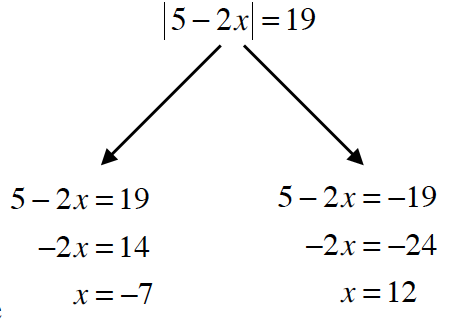### Home > CCA > Chapter 3 > Lesson 3.3.2 > Problem3-99

3-99.

One way to solve absolute value equations is to think about “looking inside” the absolute value. The “inside” must be positive or negative, so you should solve the equation both ways. For example, you could record your steps as shown at right.

Solve each equation. Be sure to find all possible answers and check your solutions. Homework Help ✎1. $|9+3x|=39$

• Create two equations, one positive, one negative.
$9+3x=39$
$9+3x=−39$
Then solve each for $x$.

$x=10$ or $x=−16$

1. $|2x+1|=10$

• See the help for part (a).

$x=\frac{9}{2},\text{ or }x= -\frac{11}{2}$

1. $|-3x+9|=10$

• See the help for part (a).

1. $|3.2x-4|=-5.7$

• No solution. Explain why.